Next: Method of Images Up: Two-Dimensional Potential Flow Previous: Complex Velocity Potential

# Complex Velocity

Equations (5.17), (5.20), and (6.15) imply that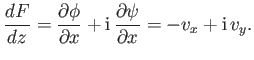(6.35)

Consequently,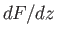is termed the complex velocity. It follows that(6.36)

where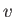is the flow speed.

A stagnation point is defined as a point in a flow pattern at which the flow speed,, falls to zero. (See Section 5.8.) According to the previous expression,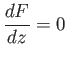(6.37)

at a stagnation point. For instance, the stagnation points of the flow pattern produced when a cylindrical obstacle of radius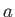, centered on the origin, is placed in a uniform flow of speed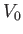, directed parallel to the-axis, and the circulation of the flow around is cylinder is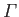, are found by setting the derivative of the complex potential (6.32) to zero. It follows that the stagnation points satisfy the quadratic equation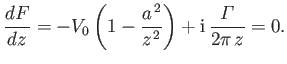(6.38)

The solutions are(6.39)

where, with the proviso that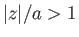, because the regionis occupied by the cylinder. Thus, if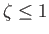then there are two stagnation points on the surface of the cylinder atand. On the other hand, if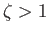then there is a single stagnation point below the cylinder at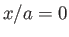and.

According to Section 4.15, Bernoulli's theorem in an steady, irrotational, incompressible fluid takes the form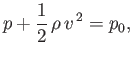(6.40)

where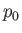is a uniform constant. Here, gravity (and any other body force) has been neglected. Thus, the pressure distribution in such a fluid can be written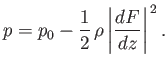(6.41)Next: Method of Images Up: Two-Dimensional Potential Flow Previous: Complex Velocity Potential
Richard Fitzpatrick 2016-03-31